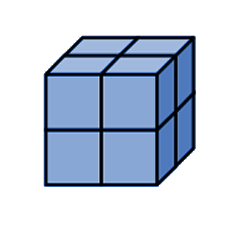# 5th Grade : Measurement and Data Standards#### The standards described below are those specific to the geometry and measurement.  These standards, generally, are pretty straightforward and similar to the more traditional standards that parents may be familiar with.#### A.  Convert like measurement units within a given measurement system.

1. Convert among different-sized standard measurement units within a given measurement system (e.g., convert 5 cm to 0.05 m), and use these conversions in solving multi-step, real world problems.

In this standard, the students may be provided a problem such as:

Johnny purchased 16 quarts of milk at the local grocery.  How many gallon containers of milk would he have to purchase to get the same amount?

#### B. Represent and interpret data.

2.  Make a line plot to display a data set of measurements in fractions of a unit (1/2, 1/4, 1/8). Use operations on fractions for this grade to solve problems involving information presented in line plots.

A line plot is a number line with the number of occurrences of each unit in a data set.  Example :From the data set, the student will solve problems related to the data.

• If the line plot above represents the height of bean plants after 3 days, what is the difference between the shortest and tallest bean plants height?
• What is the total of the the heights of the bean plants?
• Students will also be expected to collect data and represent that data on a line plot.

#### C. Geometric measurement: understand concepts of volume.

3.  Recognize volume as an attribute of solid figures and understand concepts of volume measurement.

A.  This part of this standard requires that students understand a ‘unit cube’.  Students should recognize that volume is represented by cubic units and that a unit cube is a three dimensional measurement unit that is one unit of height, width and depth.

B. Volume represents the number of ‘cubic units’ that will ‘fill’ the space without gaps or overlaps.

4.   Measure volumes by counting unit cubes, using cubic cm, cubic in, cubic ft, and improvised units.

With this standard, students should be able to measure the volume when given a visual representation and counting the unit cubes.  The students should be able to ‘count’ even those cubes that they can not see in the representation.5.  Relate volume to the operations of multiplication and addition and solve real world and mathematical problems involving volume.

A)

Find the volume of a right rectangular prism by packing it with unit cubes, and then show that the volume is the same as would be found by multiplying the edge lengths, OR  by multiplying the height by the area of the base. Represent threefold whole-number products as volumes, e.g., to represent the associative property of multiplication.

In this part of the standard, using only rectangular prisms – boxes, the students are expected to show that volume is equal to the area of the base of the structure times the height and is equal to multiplying the edge lengths (height, length and width).  The students will be expected to prove that length x height x width will result in the number of cubes it takes to ‘fill the structure’ and represent volume.  Additionally, the students should be able to explain why the order of the multiplication of the edges (length, width, height) does not matter, the volume will be the same (associative property).

B)

Apply the formulas V = l × w × h and V = b × h for rectangular prisms to find volumes of right rectangular prisms with whole-number edge lengths in the context of solving real world and mathematical problems.

With the understandings of part A of the standard, the students should be able to use the formula l x w x h to find the volume of rectangular prisms.

For example:  Johnny is given a box of cereal and asked to determine the volume of the box.  He measures the height of the box and finds that it is 10 inches tall.  He measures the width of the box and finds that it is 6 inches wide. If the box measures 2 inches deep, what is the volume of the box?C)

Recognize volume as additive. Find volumes of solid figures composed of two non-overlapping right rectangular prisms by adding the volumes of the non-overlapping parts, applying this technique to solve real world problems.

With this part of the standard, the students should be able to understand that some solid figures are made of multiple parts each with a different volume that may be added together to find the total volume.  For example, the volume of the figure to the right could be calculated by adding two parts together.  There are multiple ways to add them together, but the volume will be the same regardless. (2 x 2 x 4) added to (3 x 4 x 1)  OR (5 x 4 x 1) added to (2 x 1 x 4)

This standard includes a lot of skills and the students should have amply opportunity to practice this standard.  The good news is, it is relatively straight-forward and most parents will understand the tasks given in this standard.